# Implementing a jump with a given duration and height

DISCLAIMER: sorry for the ugly formulas, I’ll work on integrating LaTeX to my blog and update this post to make them look better.

This will be an oddly specific blog post, but I hope it can be of use to someone.

Some time ago, a friend of mine asked me for a little help on a gamedev problem. The problem was this: given a “kinematic” character, how do I make it jump such that it will reach height H and land in time T?

This is slightly different from the usual approach of character movement, where usually one defines the jump force (or velocity) and the gravity affecting the character. Here we want to derive those variables from our own conditions: the jump must last exactly T seconds and reach a height of exactly H. (Where “exactly” here actually means “must be as close as possible to”).

This problem can be solved in 2 ways. One way is to use the equation of motion: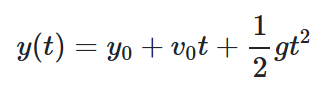We compute the equation for t = T/2 and for t = T, imposing that y is H in the first case and 0 in the second case. We also impose that y0 is 0 since we’re starting the jump from ground level (we may actually be starting from any height, but all we want from our jump is that it requires time T to be back to the starting height, so we can just set our reference frame so that the starting height is 0):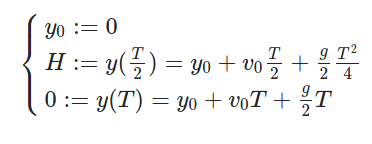Then we solve the system for v0 and g. This yields the solution we’re looking for: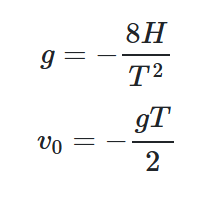### Alternative approach

Another way to approach this problem is not starting from the already-derived equation of motion, but from its parent: the differential equation y’’ = a. Then, all we need to do is adding the boundary conditions that encode our requirements and solve it.

The first boundary condition is the fact that we are defining the starting height as our y = 0.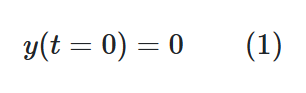Second, we want to be back to the ground level at time T: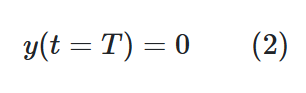The system consisting of the differential equation y’‘(t) = a, (1) and (2) gives us a family of parabulas as its solution: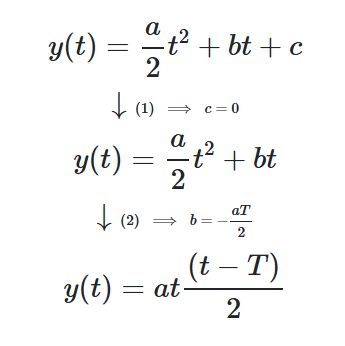Now, to find a, we need to add another boundary condition that encodes our request that the jump height is H: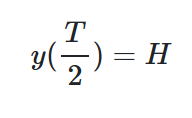We know that T/2 is the time where the jump is at its apex because y(t) is a parabula, so we impose that that point is at y = H. This gives us: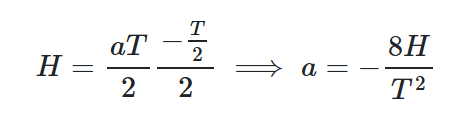Since a represents our gravity, let’s rename it g. We can now derive the starting jump speed v0 by using the primitive of our initial differential equation: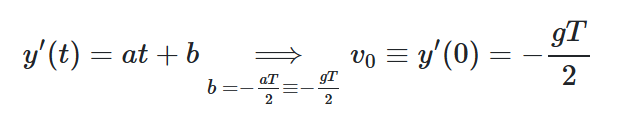So, we have derived the same solutions as above:### Godot example

In my friend’s case, she was implementing her kinematic character using the Godot Engine. We can translate the theory above in GDscript form like this:

``````class_name MyCharacter
extends KinematicBody2D

export var jump_duration: float  # T
export var jump_height: float    # H

var velocity: Vector2
var gravity: float

# Compute the gravity (g) once here
gravity = 8 * (jump_height / pow(jump_duration, 2))

func _physics_process(delta: float):
# Nothing interesting here, just update the velocity and move the character
velocity.y += gravity * delta
velocity = move_and_slide(velocity, Vector2(0, 1))

func _input(event: InputEvent):
if event.is_action_pressed("jump"):
# Set the initial jump velocity (v0) here
velocity.y -= gravity * 0.5 * jump_duration
``````

This will work as expected as long as the duration and height parameters are within “safe” ranges. If the product `T * H` gets too high, v0 may become too big for the physics simulation to properly handle. If you try and use this technique in your game, test it with different values and find how far can you take it before relying too heavily on it.

Tags:

Updated: